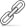9月21日下午，四川省第四届全民健身运动会残疾人柔力球比赛圆满收官。...[详情]
• []
• []
• []
• []
• []
• []
• []
• []
• []
• []
• []
• []
• []
• []
• []
• []
• []
• []
• []
• []
• []
• []
• []
• []
• []
• []
• []
• []
• []
• []
• []
• []
• []
• []
• []
• []
• []
• []

• []
• []
• []
• []
• []
• []
• []
 [] [] [] [] [] []
• []
• []
• []
• []
• []
• []
• []友情链接：
 广元市残疾人联合会版权所有蜀ICP备09004036号-1川公网安备 51080002000208号 地址：广元市东坝兴安路459号电话：0839-2301201